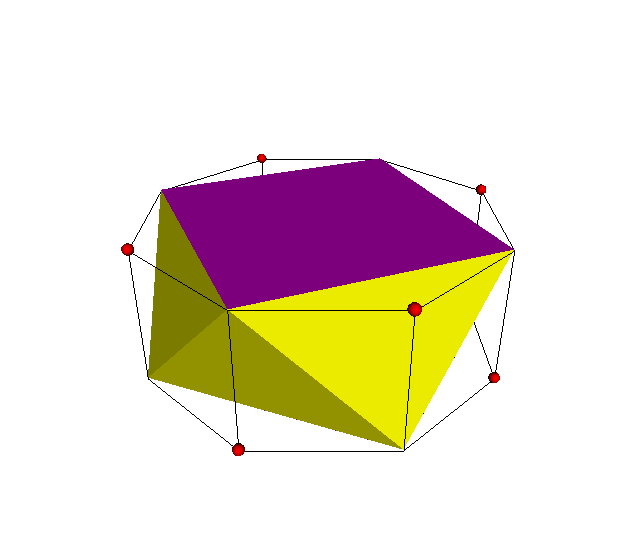Acronym n-ap Name n-gonal antiprism `© ©` Circumradius sqrt[(3-2 cos(π/n))/(8-8 cos(π/n))] Height sqrt[(1+2 cos(π/n))/(2+2 cos(π/n))] Coordinates (cos(k π/n)/[2 sin(π/n)], sin(k π/n)/[2 sin(π/n)], (-1)k h/2)   all k integral where h is the height given above Vertex figure [33,n] Snub derivation `` General of army (is itself convex) Colonel of regiment (is itself locally convex) Especially tet (n=2)*   oct (n=3)   squap (n=4)   pap (n=5)   hap (n=6)   oap (n=8)   dap (n=10)   azap (n=∞) Dihedral angles between {3} and {3}:   arccos[(1-4 cos(π/n))/3] between {3} and {n}:   arccos(-sqrt[(1-cos(π/n))/(3+3 cos(π/n))]) Confer more general: n/2-ap   n/d-ap   general polytopal classes: segmentohedra Externallinks* The case n=2 equally would be considerable here by concept, it just has a different incidence matrix as the n-gons become degenerate.

The compound of 2 mutually gyrated n-gonal antiprisms, one considered as xo-n-ox&#x, the other as ox-n-xo&#x, has for encasing convex hull a variant of the 2n-gonal prism, in fact the variant a-2n-o b, where a = 1/[2 cos(π/2n)] and b = sqrt[1 - 1/[2 cos(π/2n)]2] = sqrt[(1+2 cos(π/n))/(2+2 cos(π/n))]. This is how the below mentioned semiation as a vertex alternation (snubbing) truely works (when resizing would be considered first).

Incidence matrix according to Dynkin symbol

```s2sns   (n>2)

demi( . . .  ) | 2n | 1 1  2 | 1  3
---------------+----+--------+-----
s2s .    |  2 | n *  * | 0  2
s . s2*a |  2 | * n  * | 0  2
sefa( . sns  ) |  2 | * * 2n | 1  1
---------------+----+--------+-----
. sns    ♦  n | 0 0  n | 2  *
sefa( s2sns  ) |  3 | 1 1  1 | * 2n

starting figure: x xnx
```

```s2s2no   (n>2)

demi( . .  . ) | 2n |  2  2 | 1  3
---------------+----+-------+-----
s2s  .   |  2 | 2n  * | 0  2
sefa( . s2no ) |  2 |  * 2n | 1  1
---------------+----+-------+-----
. s2no   ♦  n |  0  n | 2  *
sefa( s2s2no ) |  3 |  2  1 | * 2n

starting figure: x x2no
```

```xonox&#x   (n>2)   → height = sqrt[(1+2 cos(π/n))/(2+2 cos(π/n))]
({n} || dual {n})

o.no.    | n * | 2  2 0 | 1 2 1 0
.on.o    | * n | 0  2 2 | 0 1 2 1
---------+-----+--------+--------
x. ..    | 2 0 | n  * * | 1 1 0 0
oonoo&#x | 1 1 | * 2n * | 0 1 1 0
.. .x    | 0 2 | *  * n | 0 0 1 1
---------+-----+--------+--------
x.no.    | n 0 | n  0 0 | 1 * * *
xo ..&#x | 2 1 | 1  2 0 | * n * *
.. ox&#x | 1 2 | 0  2 1 | * * n *
.on.x    | 0 n | 0  0 n | * * * 1
```

 © 2004-2021 top of page